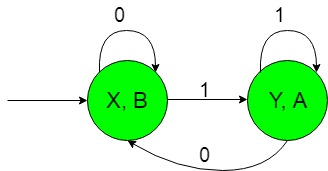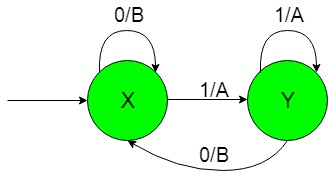# Finite Automata with Output (Set 5)

In this article, we will see some designing of Finite Automata with Output i.e, Moore and Mealy machines.
Problem: Construction of the machines that take set of all string over {0, 1} as input and produce ‘A’ as output if the input contains ‘1’ as the substring or the input string starts with ‘1’ or ends with ‘1’.
That is here we have,
Ε = {0, 1} and
Δ = {A, B}
where Ε and Δ are the input and output alphabet respectively.

The required Moore machine is constructed below:-Explanation:
In the above diagram, the initial state ‘X’ on getting ‘0’ as the input it remains in the state of itself and prints ‘B’ as the output and on getting ‘1’ as the input it transits to a state ‘Y’ and prints ‘A’ as the output. The state ‘Y’ on getting ‘1’ as the input it remains in the state of itself and prints ‘A’ as the output and on getting ‘0’ as the input it comes back to the state ‘X’ and prints ‘B’ as the output.
Thus finally above Moore machine can easily give ‘A’ as the output on getting ‘1’ as input substring.

The required Mealy machine is constructed below:-Explanation:
In the above diagram, the initial state ‘X’ on getting ‘0’ as the input it remains in the state of itself and prints ‘B’ as the output and on getting ‘1’ as the input it transits to a state ‘Y’ and prints ‘A’ as the output. The state ‘Y’ on getting ‘1’ as the input it remains in the state of itself and prints ‘A’ as the output and on getting ‘0’ as the input it comes back to the state ‘X’ and prints ‘B’ as the output.
Thus finally above Mealy machine can easily give ‘A’ as the output on getting ‘1’ as input substring.